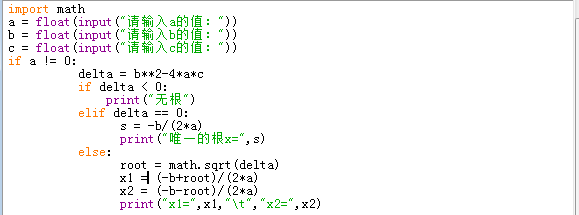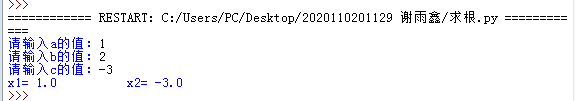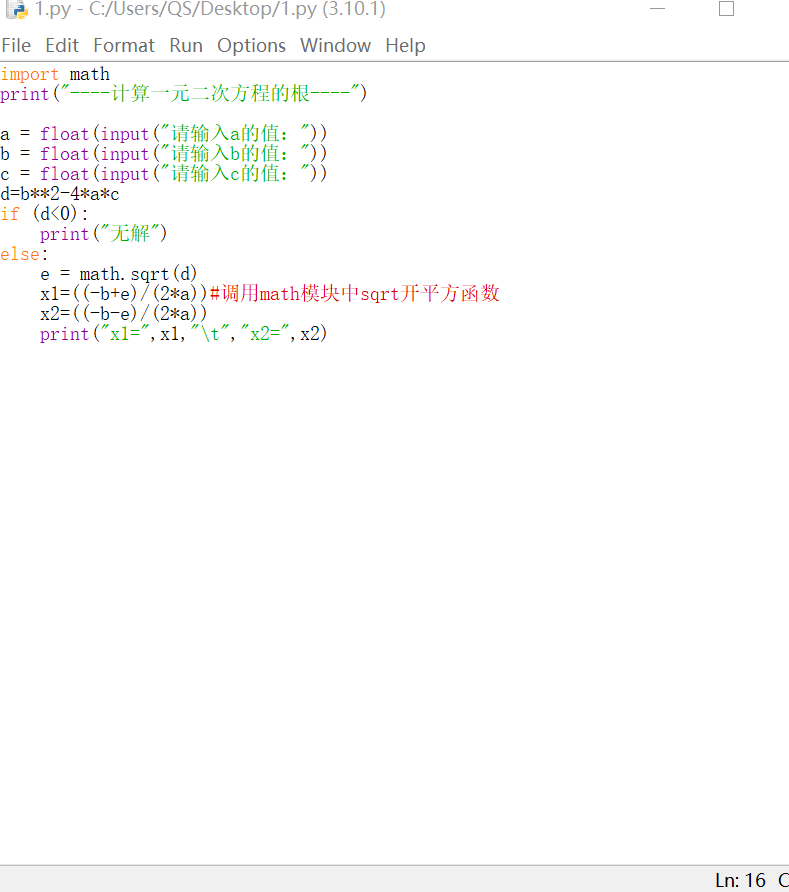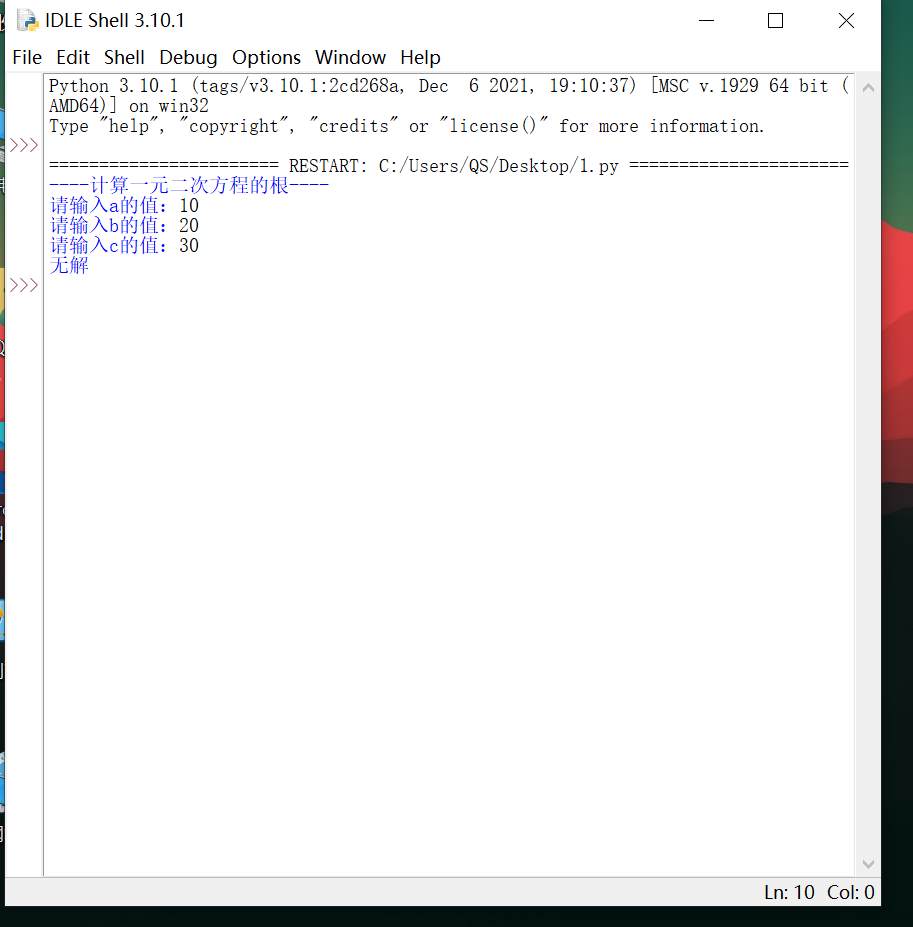• python判断一元二次方程
千次阅读
2022-05-01 10:10:15

题目：求一元二次方程ax*x+b*x+c=0的解

从键盘输入a,b,c的值，分多种情况输出解。

1. a等于0，b也等于0时，输出“方程无解”；
2. a等于0，b不等于0时，输出“方程有1个解，x= ？”；？表示方程的解
3. a不等于0时，计算判别式d=b*b-4*a*c的值：

若d小于0，输出“方程无实数解”；

若d等于0，解等于-b/2a，输出“方程有两个相同的解，x1=x2=？”；？表示方程的解

若d大于0，用求根公式 求两个解，并比较大小，使x1大于x2，输出“方程有两个实数解，          x1=?,x2=?”。?表示方程的解

代码如下：

a=float(input("请输入a"))

b=float(input("请输入b"))

c=float(input("请输入c"))

if a==0 and b==0:

print("方程无解")

elif a==0 and b!=0:

print("方程有一个解，x=",-c/b)

elif a!=0:

d=b*b-4*a*c

if d==0:

print("方程有一个解，x1=x2=",-b/(2*a))

elif d<0:

print("方程无实数解")

else:

e=b*b-4*a*c

x1=(-b+e**0.5)/(2*a)

x2=(-b-e**0.5)/(2*a)

if x1>x2:

print("方程有两个实数解，x1=%.1f,x2=%.1f"%(x1,x2))

else:

print("方程有两个实数解，x1=%.1f,x2=%.1f"%(x2,x1))

这道题就很简单了就是对输入的a,b,c进行if操作去选择它们应该执行的操作。整体的思路就是只用到了选择语句最多就是选择里面再加上了一个选择，是一个比较简单的问题。这个特别适合初学者在掌握选择操作时学习，这个是很经典的选择操作的语句代码之一。

Tips:python里面开根号可以 变量**0.5当然仅限于变量是正数，这样就不用调用math库和sqrt函数了。python
更多相关内容
• 首先如图所示敲出下列代码： 即如下 import math a = float(input("请输入a的值：")) b = float(input("请输入b的值：")) c = float(input("请输入c的值："))if a !... delta = b**2-4*a*c ... s ...

首先如图所示敲出下列代码：即如下

import math
a = float(input("请输入a的值："))
b = float(input("请输入b的值："))
c = float(input("请输入c的值："))
if a != 0:
delta = b**2-4*a*c
if delta < 0:
print("无根")
elif delta == 0:
s = -b/(2*a)
print("唯一的根x=",s)
else:
root = math.sqrt(delta)
x1 = (-b+root)/(2*a)
x2 = (-b-root)/(2*a)
print("x1=",x1,"\t","x2=",x2)

输入3个数测试，得到如图所示，即为成功搬走，不客气！

展开全文python
• 本文实例讲述了Python编程实现数学运算求一元二次方程的实根算法。分享给大家供大家参考，具体如下： 问题： 请定义一个函数quadratic(a, b, c)，接收3个参数，返回一元二次方程：ax² + bx + c = 0的两个解。 实现...
• 代码 运行结果

代码运行结果展开全文python
• python学习 1

#python一元二次方程求解

# bglei@nuaa.edu.cn 2022年5月11日
import math
z=input('输入一元二次方程系数：').split()
a,b,c=float(z),float(z),float(z)
if b**2==4*a*c:
x= (-b + math.sqrt(b*b-4*a*c))/(2*a)
print('x1=x2=%.5f' %x)
elif b**2>4*a*c:
print('x1=%.5f;x2=%.5f' %((-b + math.sqrt(b*b-4*a*c))/(2*a),(-b - math.sqrt(b*b-4*a*c))/(2*a)))
else:

re= -b / (2*a)
if abs(re-0)<0.000001:
re=0.00000
im=math.sqrt(4*a*c-b*b) / (2*a)
print('x1=%.5f+%.5fi;x2=%.5f-%.5fi' %(re,im,re,im))


运行示例：

输入一元二次方程系数：1 3 4
x1=-1.50000+1.32288i;x2=-1.50000-1.32288i

展开全文python 机器学习
• 主要介绍了Python实现求解一元二次方程的方法,涉及Python基于math包进行数值运算相关操作技巧,需要的朋友可以参考下
•python
• 最近在做笔试题的时候遇到的一道题，挺有意思的贴出来和大佬讨论一下（常规的做法，...考虑到给定的方程需要化简，因此第一步要化简等号左右两端的方程，并获取系数，并存放在字典中，移到等式一端，之后合并字典则6x-python 字符串
• Python求解一元二次方程问题【简单易懂，注释超全，代码可以直接运行】 业务需求: 解一元二次方程是初中数学中的基本知识，- -般来讲解法有公式法、因式分解法等。可以根据自己 的理解，写- -段求解一元二次方程的...python
•python
• 一元二次方程的定义是：ax² + bx + c = 0 Python的math包提供了sqrt()函数用于计算平方根。 # coding:utf-8 import math def func(a, b, c): d = b**2 - 4 * a *c if d &gt;= 0: num = math.sqrt(d) ...python
• 这是一个简单的求解一元二次方程组的python代码，作为新手的第一个完整的代码，还是有很多不足之处python
• python解一元二次方程一元二次方程的公式开始编程 一元二次方程的公式 一元二次方程的一般式为ax^2+bx+c=0（a不等于0） 一元二次方程的解法有几种，最简便的是公式法，即=b±根号b^2-4ac除以2a 开始编程 a=int...python
•python
• 本文使用Python实现一元二次方程求根公式，主要演示运算符和几个内置函数的用法，封面图片与本文内容无关。def root(a, b, c, highmiddle=True): #首...python 人工智能 机器学习
• #一元二次方程 a,b,c=float(input('a')),float(input('b')),float(input('c')) m=(b**2-4*a*c)**0.5 if m>=0: print((m-b)/2/a,(-m-b)/2/a) 无实数根时报错 ValueError: negative number cannot be raised to a...
• python一元次方程 # 方程求解 import sympy as sy import sympy import sympy as sp # 导入sympy包 import math a=math.pi #-----------------方法一---------------------- x = sp.Symbol('x') # 定义符号变量...python
• Python算法作业】解一元二次方程# 求方程ax^2+bx+c=0的根# 用到 math.sqrt()import math# solveEquationdef solveEquation(a, b, c):print("您输入的方程是：")print("{} * x^2 + {} * x + {} = 0 ".format(a, b, ...
•python
• 这里使用的是一元二次方程的求根公式，{:.2f}是把结果变为有两位小数的数字，这样看上去更加简便一点 这是运行出来的结果，希望这些内容能帮助到你们。python
• =0:break if b**2-4*a*c("该方程没有实根") else: x1=(-b+sqrt(b**2-4*a*c))/(2*a) x2=(-b-sqrt(b**2-4*a*c))/(2*a) print("x1=%6.2f\nx2=%6.2f"%(x1,x2)) 那个0是我随意输入后，系统报错的，b^2-4ac，看来编写这本...
• ## python计算一元二次方程的根

万次阅读 多人点赞 2019-06-07 11:35:16
python计算一元二次方程的根 小编也是一个小白，，只因突然想起写个求解方程，所以写了一个求解一元二次方程的代码。 发现使用python写求解方程要比c语言简单。。但是学艺不精，，勿喷 以下代码能够实现有解的...
•python
• Python实现求解一元二次方程的方法示例本文实例讲述了Python实现求解一元二次方程的方法。分享给大家供大家参考，具体如下：1. 引入math包2. 定义返回的对象3. 判断b*b-4ac的大小具体计算代码如下：# -*- coding:utf...
• 计算机语言运用--数值计算9-方程的计算机处理92-4_Fortran数值计算方程的计算机处理这里说的方程，是以实数域为计算范围。这里说的方程：是含有未知数的等式。可以是a+5=8，或X+A=B，或ax2+bx+c=0，或ax+by=c与dx-ey......